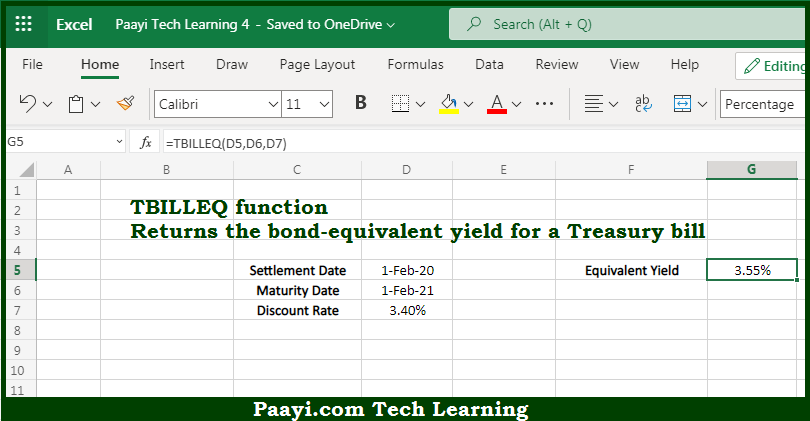# Learn How to Use Microsoft Excel TBILLEQ Function

Written by | 0 Comments | 469 Views

In this article, you will learn how to use the Microsoft Excel TBILLEQ function and its prime function in Microsoft Excel. You will also get to know the Microsoft Excel TBILLEQ function return value and syntax with the help of some examples.

Microsoft Excel TBILLEQ Function

The main purpose of the Microsoft Excel TBILLEQ function is to get the bond equivalent yield for the treasury bill. That implies, with the help of the TBILLEQ function you can able to return the bond-equivalent yield for a Treasury bill. So, with the help of the TBILLEQ function, you can able to get the bond equivalent yield for the treasury bill.

Return Value of TBILLEQ Function

The return value will be the yield in the form of a percentage.

Syntax of TBILLEQ Function

=TBILLEQ(settlement, maturity, discount)

Where the arguments:

• settlement: This is the settlement date of the security.
• maturity: This is the maturity date of the security.
• discount: This is the discount rate of the security.

How to Use Microsoft Excel TBILLEQ Function?So we know that Microsoft Excel TBILLEQ function you can able to get the bond equivalent yield for the treasury bill. That implies, with the help of the TBILLEQ function you can able to return the bond-equivalent yield for a Treasury bill. So, with the help of the TBILLEQ function, you can able to get the bond equivalent yield for the treasury bill.